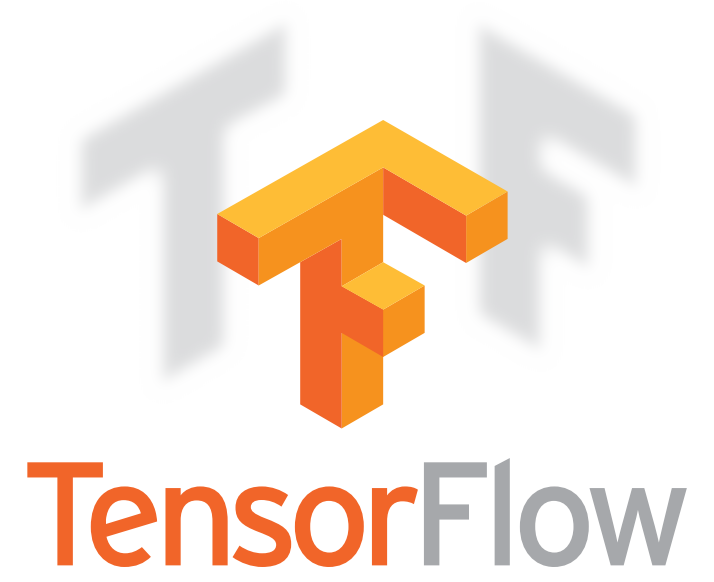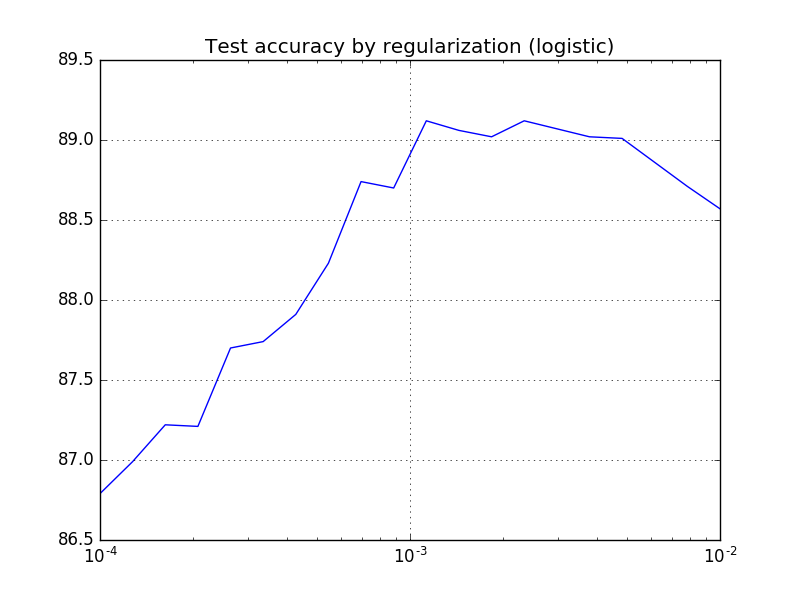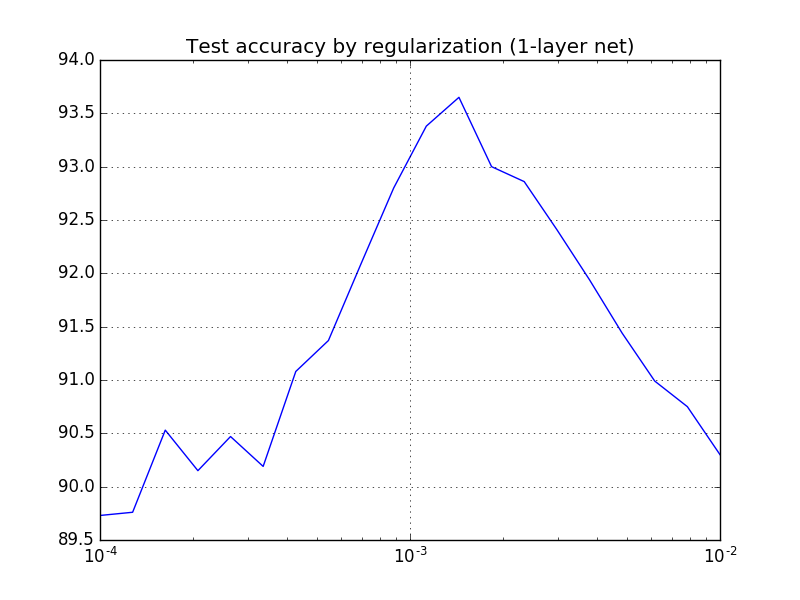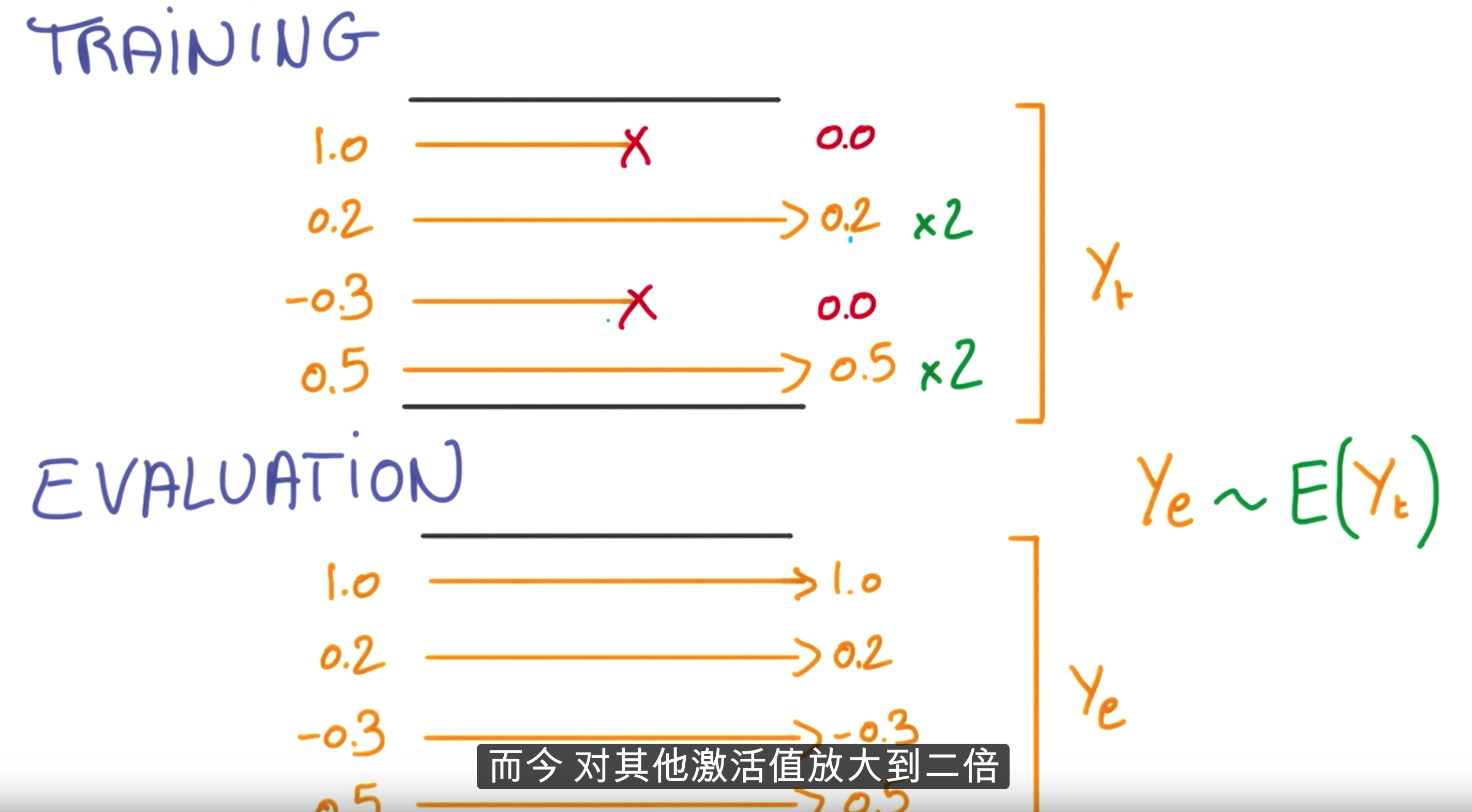# 谷歌深度学习公开课任务 3: 正则化## 题目1

### 逻辑斯谛回归

```beta_val = np.logspace(-4, -2, 20)
accuracy_val = []

# logistic model
batch_size = 128

graph = tf.Graph()
with graph.as_default():
# Input data. For the training data, we use a placeholder that will be fed
# at run time with a training minibatch.
tf_train_dataset = tf.placeholder(tf.float32,
shape=(batch_size, image_size * image_size))
tf_train_labels = tf.placeholder(tf.float32, shape=(batch_size, num_labels))
tf_valid_dataset = tf.constant(valid_dataset)
tf_test_dataset = tf.constant(test_dataset)
beta_regul = tf.placeholder(tf.float32)

# Variables.
weights = tf.Variable(tf.truncated_normal([image_size * image_size, num_labels]))
biases = tf.Variable(tf.zeros([num_labels]))

# Training computation.
logits = tf.matmul(tf_train_dataset, weights) + biases
loss = tf.reduce_mean(
tf.nn.softmax_cross_entropy_with_logits(logits, tf_train_labels)) + beta_regul * tf.nn.l2_loss(weights)

# Optimizer.

# Predictions for the training, validation, and test data.
train_prediction = tf.nn.softmax(logits)
valid_prediction = tf.nn.softmax(tf.matmul(tf_valid_dataset, weights) + biases)
test_prediction = tf.nn.softmax(tf.matmul(tf_test_dataset, weights) + biases)

num_steps = 3001

for beta in beta_val:
with tf.Session(graph=graph) as session:
tf.initialize_all_variables().run()
for step in range(num_steps):
# Pick an offset within the training data, which has been randomized.
# Note: we could use better randomization across epochs.
offset = (step * batch_size) % (train_labels.shape - batch_size)
# Generate a minibatch.
batch_data = train_dataset[offset:(offset + batch_size), :]
batch_labels = train_labels[offset:(offset + batch_size), :]
# Prepare a dictionary telling the session where to feed the minibatch.
# The key of the dictionary is the placeholder node of the graph to be fed,
# and the value is the numpy array to feed to it.
feed_dict = {tf_train_dataset: batch_data, tf_train_labels: batch_labels, beta_regul: beta}
_, l, predictions = session.run([optimizer, loss, train_prediction], feed_dict=feed_dict)
# if (step % 500 == 0):
#     print("Minibatch loss at step %d: %f" % (step, l))
#     print("Minibatch accuracy: %.1f%%" % accuracy(predictions, batch_labels))
#     print("Validation accuracy: %.1f%%" % accuracy(valid_prediction.eval(), valid_labels))
print("L2 regularization(beta=%.5f) Test accuracy: %.1f%%" % (
beta, accuracy(test_prediction.eval(), test_labels)))

accuracy_val.append(accuracy(test_prediction.eval(), test_labels))

print('Best beta=%f, accuracy=%.1f%%' % (beta_val[np.argmax(accuracy_val)], max(accuracy_val)))
plt.semilogx(beta_val, accuracy_val)
plt.grid(True)
plt.title('Test accuracy by regularization (logistic)')
plt.show()```

```L2 regularization(beta=0.00010) Test accuracy: 86.8%
L2 regularization(beta=0.00013) Test accuracy: 87.0%
L2 regularization(beta=0.00016) Test accuracy: 87.2%
L2 regularization(beta=0.00021) Test accuracy: 87.2%
L2 regularization(beta=0.00026) Test accuracy: 87.7%
L2 regularization(beta=0.00034) Test accuracy: 87.7%
L2 regularization(beta=0.00043) Test accuracy: 87.9%
L2 regularization(beta=0.00055) Test accuracy: 88.2%
L2 regularization(beta=0.00070) Test accuracy: 88.7%
L2 regularization(beta=0.00089) Test accuracy: 88.7%
L2 regularization(beta=0.00113) Test accuracy: 89.1%
L2 regularization(beta=0.00144) Test accuracy: 89.1%
L2 regularization(beta=0.00183) Test accuracy: 89.0%
L2 regularization(beta=0.00234) Test accuracy: 89.1%
L2 regularization(beta=0.00298) Test accuracy: 89.1%
L2 regularization(beta=0.00379) Test accuracy: 89.0%
L2 regularization(beta=0.00483) Test accuracy: 89.0%
L2 regularization(beta=0.00616) Test accuracy: 88.9%
L2 regularization(beta=0.00785) Test accuracy: 88.7%
L2 regularization(beta=0.01000) Test accuracy: 88.6%
Best beta=0.001129, accuracy=89.1%```### 神经网络模型

```# NN model
batch_size = 128
hidden_size = 1024

graph = tf.Graph()
with graph.as_default():
# Input data. For the training data, we use a placeholder that will be fed
# at run time with a training minibatch.
tf_train_dataset = tf.placeholder(tf.float32, shape=(batch_size, image_size * image_size))
tf_train_labels = tf.placeholder(tf.float32, shape=(batch_size, num_labels))
tf_valid_dataset = tf.constant(valid_dataset)
tf_test_dataset = tf.constant(test_dataset)
tf_beta = tf.placeholder(tf.float32)

# Variables.
W1 = tf.Variable(tf.truncated_normal([image_size * image_size, hidden_size]))
b1 = tf.Variable(tf.zeros([hidden_size]))

W2 = tf.Variable(tf.truncated_normal([hidden_size, num_labels]))
b2 = tf.Variable(tf.zeros([num_labels]))

# Training computation.
y1 = tf.nn.relu(tf.matmul(tf_train_dataset, W1) + b1)
logits = tf.matmul(y1, W2) + b2

loss = tf.reduce_mean(tf.nn.softmax_cross_entropy_with_logits(logits, tf_train_labels))

loss = loss + tf_beta * (tf.nn.l2_loss(W1) + tf.nn.l2_loss(b1) + tf.nn.l2_loss(W2) + tf.nn.l2_loss(b2))

# Optimizer.

# Predictions for the training, validation, and test data.
train_prediction = tf.nn.softmax(logits)

y1_valid = tf.nn.relu(tf.matmul(tf_valid_dataset, W1) + b1)
valid_logits = tf.matmul(y1_valid, W2) + b2
valid_prediction = tf.nn.softmax(valid_logits)

y1_test = tf.nn.relu(tf.matmul(tf_test_dataset, W1) + b1)
test_logits = tf.matmul(y1_test, W2) + b2
test_prediction = tf.nn.softmax(test_logits)

# Let's run it:
num_steps = 3001

accuracy_val.clear()
for beta in np.logspace(-4, -2, 20):
with tf.Session(graph=graph) as session:
tf.initialize_all_variables().run()
# print("Initialized")
for step in range(num_steps):
# Pick an offset within the training data, which has been randomized.
# Note: we could use better randomization across epochs.
offset = (step * batch_size) % (train_labels.shape - batch_size)
# Generate a minibatch.
batch_data = train_dataset[offset:(offset + batch_size), :]
batch_labels = train_labels[offset:(offset + batch_size), :]
# Prepare a dictionary telling the session where to feed the minibatch.
# The key of the dictionary is the placeholder node of the graph to be fed,
# and the value is the numpy array to feed to it.
feed_dict = {tf_train_dataset: batch_data, tf_train_labels: batch_labels, tf_beta: beta}
_, l, predictions = session.run([optimizer, loss, train_prediction], feed_dict=feed_dict)
# if (step % 500 == 0):
#     print("Minibatch loss at step %d: %f" % (step, l))
#     print("Minibatch accuracy: %.1f%%" % accuracy(predictions, batch_labels))
#     print("Validation accuracy: %.1f%%" % accuracy(valid_prediction.eval(), valid_labels))
print("L2 regularization(beta=%.5f) Test accuracy: %.1f%%" % (
beta, accuracy(test_prediction.eval(), test_labels)))
accuracy_val.append(accuracy(test_prediction.eval(), test_labels))

print('Best beta=%f, accuracy=%.1f%%' % (beta_val[np.argmax(accuracy_val)], max(accuracy_val)))
plt.semilogx(beta_val, accuracy_val)
plt.grid(True)
plt.title('Test accuracy by regularization (1-layer net)')
plt.show()```

```L2 regularization(beta=0.00010) Test accuracy: 89.7%
L2 regularization(beta=0.00013) Test accuracy: 89.8%
L2 regularization(beta=0.00016) Test accuracy: 90.5%
L2 regularization(beta=0.00021) Test accuracy: 90.2%
L2 regularization(beta=0.00026) Test accuracy: 90.5%
L2 regularization(beta=0.00034) Test accuracy: 90.2%
L2 regularization(beta=0.00043) Test accuracy: 91.1%
L2 regularization(beta=0.00055) Test accuracy: 91.4%
L2 regularization(beta=0.00070) Test accuracy: 92.1%
L2 regularization(beta=0.00089) Test accuracy: 92.8%
L2 regularization(beta=0.00113) Test accuracy: 93.4%
L2 regularization(beta=0.00144) Test accuracy: 93.7%
L2 regularization(beta=0.00183) Test accuracy: 93.0%
L2 regularization(beta=0.00234) Test accuracy: 92.9%
L2 regularization(beta=0.00298) Test accuracy: 92.4%
L2 regularization(beta=0.00379) Test accuracy: 91.9%
L2 regularization(beta=0.00483) Test accuracy: 91.4%
L2 regularization(beta=0.00616) Test accuracy: 91.0%
L2 regularization(beta=0.00785) Test accuracy: 90.8%
L2 regularization(beta=0.01000) Test accuracy: 90.3%
Best beta=0.001438, accuracy=93.7%```## 题目2：过拟合

```few_batch_size = batch_size * 5
small_train_dataset = train_dataset[:few_batch_size, :]
small_train_labels = train_labels[:few_batch_size, :]

print('Training set', small_train_dataset.shape, small_train_labels.shape)

num_steps = 3001

with tf.Session(graph=graph) as session:
tf.initialize_all_variables().run()
print("Initialized")
for step in range(num_steps):
# Pick an offset within the training data, which has been randomized.
# Note: we could use better randomization across epochs.
offset = (step * batch_size) % (small_train_labels.shape - batch_size)
# Generate a minibatch.
batch_data = small_train_dataset[offset:(offset + batch_size), :]
batch_labels = small_train_labels[offset:(offset + batch_size), :]
# Prepare a dictionary telling the session where to feed the minibatch.
# The key of the dictionary is the placeholder node of the graph to be fed,
# and the value is the numpy array to feed to it.
feed_dict = {tf_train_dataset: batch_data, tf_train_labels: batch_labels, tf_beta: 0.001438}
_, l, predictions = session.run(
[optimizer, loss, train_prediction], feed_dict=feed_dict)
if (step % 500 == 0):
print("Minibatch loss at step %d: %f" % (step, l))
print("Minibatch accuracy: %.1f%%" % accuracy(predictions, batch_labels))
print("Validation accuracy: %.1f%%" % accuracy(
valid_prediction.eval(), valid_labels))
print("Overfitting with small dataset Test accuracy: %.1f%%" % accuracy(test_prediction.eval(), test_labels))```

```Training set (640, 784) (640, 10)
Overfitting with small dataset Test accuracy: 84.5%```

## 题目3：Dropout

### 改善性能

```batch_size = 128
hidden_size = 1024

graph = tf.Graph()
with graph.as_default():
# Input data. For the training data, we use a placeholder that will be fed
# at run time with a training minibatch.
tf_train_dataset = tf.placeholder(tf.float32, shape=(batch_size, image_size * image_size))
tf_train_labels = tf.placeholder(tf.float32, shape=(batch_size, num_labels))
tf_valid_dataset = tf.constant(valid_dataset)
tf_test_dataset = tf.constant(test_dataset)
tf_beta = tf.placeholder(tf.float32)

# Variables.
W1 = tf.Variable(tf.truncated_normal([image_size * image_size, hidden_size]))
b1 = tf.Variable(tf.zeros([hidden_size]))

W2 = tf.Variable(tf.truncated_normal([hidden_size, num_labels]))
b2 = tf.Variable(tf.zeros([num_labels]))

# Training computation.
y1 = tf.nn.relu(tf.matmul(tf_train_dataset, W1) + b1)
y1 = tf.nn.dropout(y1, 0.5)  # Dropout
logits = tf.matmul(y1, W2) + b2

loss = tf.reduce_mean(tf.nn.softmax_cross_entropy_with_logits(logits, tf_train_labels))

loss = loss + tf_beta * (tf.nn.l2_loss(W1) + tf.nn.l2_loss(b1) + tf.nn.l2_loss(W2) + tf.nn.l2_loss(b2))

# Optimizer.

# Predictions for the training, validation, and test data.
train_prediction = tf.nn.softmax(logits)

y1_valid = tf.nn.relu(tf.matmul(tf_valid_dataset, W1) + b1)
valid_logits = tf.matmul(y1_valid, W2) + b2
valid_prediction = tf.nn.softmax(valid_logits)

y1_test = tf.nn.relu(tf.matmul(tf_test_dataset, W1) + b1)
test_logits = tf.matmul(y1_test, W2) + b2
test_prediction = tf.nn.softmax(test_logits)

# Let's run it:
num_steps = 3001

with tf.Session(graph=graph) as session:
tf.initialize_all_variables().run()
print("Initialized")
for step in range(num_steps):
# Pick an offset within the training data, which has been randomized.
# Note: we could use better randomization across epochs.
offset = (step * batch_size) % (train_labels.shape - batch_size)
# Generate a minibatch.
batch_data = train_dataset[offset:(offset + batch_size), :]
batch_labels = train_labels[offset:(offset + batch_size), :]
# Prepare a dictionary telling the session where to feed the minibatch.
# The key of the dictionary is the placeholder node of the graph to be fed,
# and the value is the numpy array to feed to it.
feed_dict = {tf_train_dataset: batch_data, tf_train_labels: batch_labels, tf_beta: 0.001438}
_, l, predictions = session.run([optimizer, loss, train_prediction], feed_dict=feed_dict)
if (step % 500 == 0):
print("Minibatch loss at step %d: %f" % (step, l))
print("Minibatch accuracy: %.1f%%" % accuracy(predictions, batch_labels))
print("Validation accuracy: %.1f%%" % accuracy(
valid_prediction.eval(), valid_labels))
print("Dropout Test accuracy: %.1f%%" % accuracy(test_prediction.eval(), test_labels))```

`y1 = tf.nn.dropout(y1, 0.5)  # Dropout`With probability keep_prob, outputs the input element scaled up by 1 / keep_prob, otherwise outputs 0. The scaling is so that the expected sum is unchanged.

`Dropout Test accuracy: 92.3%`

### 改善过拟合

`Dropout with small dataset Test accuracy: 86.1%`

## 题目4：深度神经网络

```batch_size = 128
fc1_size = 4096
fc2_size = 2048
fc3_size = 128

graph = tf.Graph()
with graph.as_default():
# Input data. For the training data, we use a placeholder that will be fed
# at run time with a training minibatch.
tf_train_dataset = tf.placeholder(tf.float32,
shape=(batch_size, image_size * image_size))
tf_train_labels = tf.placeholder(tf.float32, shape=(batch_size, num_labels))
tf_valid_dataset = tf.constant(valid_dataset)
tf_test_dataset = tf.constant(test_dataset)
tf_beta = tf.placeholder(tf.float32)
global_step = tf.Variable(0)  # count the number of steps taken.

# Variables.
# stddev is very important!!!
W1 = tf.Variable(
tf.truncated_normal([image_size * image_size, fc1_size], stddev=np.sqrt(2.0 / (image_size * image_size))))
b1 = tf.Variable(tf.zeros([fc1_size]))

W2 = tf.Variable(tf.truncated_normal([fc1_size, fc2_size], stddev=np.sqrt(2.0 / fc1_size)))
b2 = tf.Variable(tf.zeros([fc2_size]))

W3 = tf.Variable(tf.truncated_normal([fc2_size, fc3_size], stddev=np.sqrt(2.0 / fc2_size)))
b3 = tf.Variable(tf.zeros([fc3_size]))

W4 = tf.Variable(tf.truncated_normal([fc3_size, num_labels], stddev=np.sqrt(2.0 / fc3_size)))
b4 = tf.Variable(tf.zeros([num_labels]))

# Training computation.
y1 = tf.nn.relu(tf.matmul(tf_train_dataset, W1) + b1)
# y1 = tf.nn.dropout(y1, 0.5)

y2 = tf.nn.relu(tf.matmul(y1, W2) + b2)
# y2 = tf.nn.dropout(y2, 0.5)

y3 = tf.nn.relu(tf.matmul(y2, W3) + b3)
# y3 = tf.nn.dropout(y3, 0.5)

logits = tf.matmul(y3, W4) + b4

loss = tf.reduce_mean(
tf.nn.softmax_cross_entropy_with_logits(logits, tf_train_labels))

loss = loss + tf_beta * (tf.nn.l2_loss(W1) + tf.nn.l2_loss(b1) + tf.nn.l2_loss(W2) + tf.nn.l2_loss(b2) +
tf.nn.l2_loss(W3) + tf.nn.l2_loss(b3) + tf.nn.l2_loss(W4) + tf.nn.l2_loss(b4))

# Optimizer
learning_rate = tf.train.exponential_decay(0.5, global_step, 1000, 0.7, staircase=True)

# Predictions for the training, validation, and test data.
train_prediction = tf.nn.softmax(logits)

y1_valid = tf.nn.relu(tf.matmul(tf_valid_dataset, W1) + b1)
y2_valid = tf.nn.relu(tf.matmul(y1_valid, W2) + b2)
y3_valid = tf.nn.relu(tf.matmul(y2_valid, W3) + b3)
valid_logits = tf.matmul(y3_valid, W4) + b4
valid_prediction = tf.nn.softmax(valid_logits)

y1_test = tf.nn.relu(tf.matmul(tf_test_dataset, W1) + b1)
y2_test = tf.nn.relu(tf.matmul(y1_test, W2) + b2)
y3_test = tf.nn.relu(tf.matmul(y2_test, W3) + b3)
test_logits = tf.matmul(y3_test, W4) + b4
test_prediction = tf.nn.softmax(test_logits)

# Let's run it:
num_steps = 12001

with tf.Session(graph=graph) as session:
tf.initialize_all_variables().run()
print("Initialized")
for step in range(num_steps):
# Pick an offset within the training data, which has been randomized.
# Note: we could use better randomization across epochs.
offset = (step * batch_size) % (train_labels.shape - batch_size)
# Generate a minibatch.
batch_data = train_dataset[offset:(offset + batch_size), :]
batch_labels = train_labels[offset:(offset + batch_size), :]
# Prepare a dictionary telling the session where to feed the minibatch.
# The key of the dictionary is the placeholder node of the graph to be fed,
# and the value is the numpy array to feed to it.
feed_dict = {tf_train_dataset: batch_data, tf_train_labels: batch_labels, tf_beta: 0.001438}
_, l, predictions = session.run([optimizer, loss, train_prediction], feed_dict=feed_dict)
if (step % 500 == 0):
print("Minibatch loss at step %d: %f" % (step, l))
print("Minibatch accuracy: %.1f%%" % accuracy(predictions, batch_labels))
print("Validation accuracy: %.1f%%" % accuracy(
valid_prediction.eval(), valid_labels))
print("Final Test accuracy: %.1f%%" % accuracy(test_prediction.eval(), test_labels))```

`learning_rate = tf.train.exponential_decay(0.5, global_step, 1000, 0.7, staircase=True)`

MBP烧开两壶水之后输出：

`Final Test accuracy: 96.0%`

## Reference

https://github.com/Arn-O/udacity-deep-learning

https://github.com/runhani/udacity-deep-learning